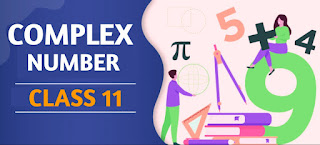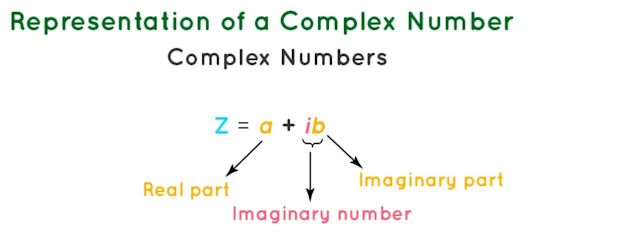# Complex Numbers Class 11 Mathematics Solutions | Exercise - 7.1## Chapter - 7  Complex Numbers

A complex number can be defined as a number that can be expressed in the form ( a + ib ), where a and b are real numbers and 'i' is the imaginary unit, which is defined as the square root of -1. Here, the real part of a complex number a + ib is a, and the imaginary part is b. Complex numbers can be easily added, subtracted, multiplied and divided using simple rules.

In this chapter, we will learn the concept of complex numbers and their properties. In addition that, we will also learn the basic operations like addition, subtraction, multiplication, and division of complex numbers. Before, we move forward it is better to learn some terms and important formulas related to complex number. So let's have a brief introduction of the complex number chapter.

### Introduction

Earlier in the text we discussed about the set of real numbers. We have also seen how real numbers can be represented by points in a straight line, called the real line or the number line. Every real number corresponds to a point in the real line and conversely. One important fact about real number is

"The square of a real number is never negative."

A problem occurs when we solve the equation of the type x² + 4 = 0 Because there is no value of x in the real number system that the square of x is negative. In order to overcome this difficulty, we have to extend the system of numbers. This is done by introducing new set of numbers called complex numbers. It is believed that Cardon used complex numbers in 1546.

### Complex Numbers

An ordered pair of real numbers is defined to be a Complex Number. Thus, if a and b are real numbers, the ordered pair written as (a, b) is a complex number. The first number, a is called the real part and the second number, b the imaginary part of the complex number.

Since each ordered pair can be located by a point in the plane so, the complex number (a, b) can be located by a point in the plane. The real part a taken along the x-axis is known as the real axis and the imaginary part b, taken along the y-axis is known as the imaginary axis. The plane in which the complex number is plotted is known as the complex plane.

A complex number is usually denoted by a single letter such as z, w etc. If z = (a, b) is a complex number, the real and the imaginary parts of z are respectively denoted by Re(z) and Im(z) . Thus Re(z) = a and Im(z) = b. Two complex numbers (a, b) and (c, d) are said to be equal if and only if a = c and b = d.

### The Imaginary Unit

We have seen that the complex number (a, 0) plays the same role as the real number a Hence the real number a can be treated as the complex number (a, 0) with the imaginary part 0. Thus the complex number (1, 0) is same as the real number 1. The complex number (0, 1) with the real part zero and the imaginary part 1 is denoted by the letter '7' (the iota in the Greek alphabet) and it is called the imaginary unit. The symbol i was introduced by Euler in 1777. The complex number (0, 1) is denoted by i and is called the imaginary unit.

Theorems

Theorem I
i² = - 1
Proof:-
i² = i.i = (0, 1)(0, 1)
= (0 - 1, 0 + 0)
= (-1,0)
= - (1,0)
= -1

Theorem II
If a and b are real numbers then the complex number (a, b) can be written as (a + ib) where i = (0, 1), and i² = -1
Proof:-
a+b = (a,0) + (0, 1) (b, 0)
= (a,0) + (0,b)
= (a, b)
So, if a and b are real numbers then (a + ib) is said to be a complex number where i² = -1.### Exercise - 7.1

In this PDF, you'll only find the solution of class 11 complex number chapter. It contains all the solutions of exercise- 7.1. If you want the solutions of other exercises then you'll find them above this PDF. Just click on the button and you'll reach your destination.

NoteScroll the PDF to view all Solution

You are not allowed to post this PDF in any website or social platform without permission.

## Is Class 11 Mathematics Guide Helpful For Student ?

I have published this Notes for helping students who can't solve difficult maths problems. Student should not fully depend on this note for completing all the exercises. If you totally depend on this note and simply copy as it is then it may affect your study.

Student should also use their own will power and try to solve problems themselves. You can use this mathematics guide PDF as a reference. You should check all the answers before copying because all the answers may not be correct. There may be some minor mistakes in the note, please consider those mistakes.

## How to secure good marks in Mathematics ?

As, you may know I'm also a student. Being a student is not so easy. You have to study different subjects simultaneously. From my point of view most of the student are weak in mathematics. You can take me as an example, I am also weak in mathematics. I also face problems while solving mathematics questions.

If you want to secure good marks in mathematics then you should practise them everyday. You should once revise all the exercise which are already taught in class. When you are solving maths problems, start from easy questions that you know already. If you do so then you won't get bored.

Maths is not only about practising, especially in grade 11 you to have the basic concept of the problem. When you get the main concept of the problem then you can easily any problems in which similar concept are applied.

When your teacher tries to make the concept clear by giving examples then all students tries to remember the same example but you should never do that. You can create your own formula which you won't forget later.

If you give proper time for your practise with proper technique then you can definitely score a good marks in your examination.

Disclaimer: This website is made for educational purposes. If you find any content that belongs to you then contact us through the contact form. We will remove that content from our as soon as possible.

If you have any queries then feel free to comment down.

No Comment